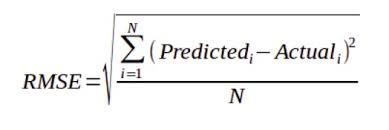Open In App
Related Articles
• Write an Interview Experience
• Machine Learning Algorithms
• Root-Mean-Square Error in R Programming

# Root-Mean-Square Error in R Programming

Root mean squared error (RMSE) is the square root of the mean of the square of all of the error. RMSE is considered an excellent general-purpose error metric for numerical predictions. RMSE is a good measure of accuracy, but only to compare prediction errors of different models or model configurations for a particular variable and not between variables, as it is scale-dependent. It is the measure of how well a regression line fits the data points. The formula for calculating RMSE is:where,
predictedi = The predicted value for the ith observation.
actuali = The observed(actual) value for the ith observation
N = Total number of observations.

Note: The difference between the actual values and the predicted values is known as residuals.

#### Implementation of RMSE

The `rmse()` function available in `Metrics` package in R is used to calculate root mean square error between actual values and predicted values.

Syntax:
rmse(actual, predicted)

Parameters:
actual: The ground truth numeric vector.
predicted: The predicted numeric vector, where each element in the vector is a prediction for the corresponding element in actual.

Example 1:
Let’s define two vectors actual vector with ground truth numeric values and predicted vector with predicted numeric values where each element in the vector is a prediction for the corresponding element in actual.

 `# R program to illustrate RMSE`` ` `# Importing the required package``library(Metrics)`` ` `# Taking two vectors``actual ``=` `c(``1.5``, ``1.0``, ``2.0``, ``7.4``, ``5.8``, ``6.6``)         ``predicted ``=` `c(``1.0``, ``1.1``, ``2.5``, ``7.3``, ``6.0``, ``6.2``)      `` ` `# Calculating RMSE using rmse()         ``result ``=` `rmse(actual, predicted)`` ` `# Printing the value``print``(result)       `

Output:

``` 0.3464102
```

Example 2:
In this example let’s take the trees data in the datasets library which represents the data from a study conducted on black cherry trees.

 `# Importing required packages``library``(datasets)``library``(tidyr)``library``(dplyr)`` ` `# Access the data from R’s datasets package``data``(trees)`` ` `# Display the data in the trees dataset    ``trees           `

Output:

```    Girth Height Volume
1    8.3     70   10.3
2    8.6     65   10.3
3    8.8     63   10.2
4   10.5     72   16.4
5   10.7     81   18.8
6   10.8     83   19.7
7   11.0     66   15.6
8   11.0     75   18.2
9   11.1     80   22.6
10  11.2     75   19.9
11  11.3     79   24.2
12  11.4     76   21.0
13  11.4     76   21.4
14  11.7     69   21.3
15  12.0     75   19.1
16  12.9     74   22.2
17  12.9     85   33.8
18  13.3     86   27.4
19  13.7     71   25.7
20  13.8     64   24.9
21  14.0     78   34.5
22  14.2     80   31.7
23  14.5     74   36.3
24  16.0     72   38.3
25  16.3     77   42.6
26  17.3     81   55.4
27  17.5     82   55.7
28  17.9     80   58.3
29  18.0     80   51.5
30  18.0     80   51.0
31  20.6     87   77.0
```

 `# Look at the structure``# Of the variables``str``(trees)     `

Output:

```'data.frame':   31 obs. of  3 variables:
\$ Girth : num  8.3 8.6 8.8 10.5 10.7 10.8 11 11 11.1 11.2 ...
\$ Height: num  70 65 63 72 81 83 66 75 80 75 ...
\$ Volume: num  10.3 10.3 10.2 16.4 18.8 19.7 15.6 18.2 22.6 19.9 ...
```

This data set consists of 31 observations of 3 numeric variables describing black cherry trees with trunk girth, height and volume as variables.Now, try to fit a linear regression model to predict Volume of the trunks on the basis of given trunk girth. The Simple Liner Regression Model in R will help in this case. Let’s dive right in and build a linear model relating tree volume to girth. R makes this straightforward with the base function `lm()`. How well will the model do at predicting that tree’s volume from its girth? Use the `predict()` function, a generic R function for making predictions of model-fitting functions. `predict()` takes as arguments, the linear regression model and the values of the predictor variable that we want response variable values for.

 `# Building a linear model ``# Relating tree volume to girth``fit_1 <- ``lm``(Volume ~ Girth, data = trees)                            ``trees.Girth = trees %>% ``select``(Girth) `` ` `# Use predict function to predict volume``data.predicted = ``c``(``predict``(fit_1, ``data.frame``(Girth = trees.Girth)))    ``data.predicted`

Output:

```        1         2         3         4         5         6         7         8         9
5.103149  6.622906  7.636077 16.248033 17.261205 17.767790 18.780962 18.780962 19.287547
10        11        12        13        14        15        16        17        18
19.794133 20.300718 20.807304 20.807304 22.327061 23.846818 28.406089 28.406089 30.432431
19        20        21        22        23        24        25        26        27
32.458774 32.965360 33.978531 34.991702 36.511459 44.110244 45.630001 50.695857 51.709028
28        29        30        31
53.735371 54.241956 54.241956 67.413183
```

Now we have the actual volume of cherry tree trunks and the predicted one as driven by the linear regression models. Finally use `rmse()` function to get the relative error between the actual and the predicted values.

 `# Load the Metrics package ``library``(Metrics)`` ` `# Applying rmse() function ``rmse``(trees\$Volume, ``predict``(fit_1, ``data.frame``(Girth = trees.Girth)))`

Output:

``` 4.11254
```

As the error value is 4.11254 which is a good score for a linear model. But it can be reduced further by adding more predictors(Multiple Regression Model). So, in summary, it can be said that it is very easy to find the root mean square error using R. One can perform this task using `rmse()` function in R.